# Double mapping cylinder

(diff) ← Older revision | Latest revision (diff) | Newer revision → (diff)
Jump to: navigation, search
This article describes a construct that involves some variant of taking a product of a topological space with the unit interval and then making some identifications, typically at the endpoints, based on some specific maps.
View more such constructs

## Definition

Suppose$X,Y,Z$ are topological spaces and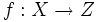$f:X \to Z$ and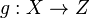$g: X \to Z$ are continuous maps. The double mapping cylinder of$f$ and$g$ is defined as the quotient of$X \times [0,1] \sqcup Y \sqcup Z$ via the relations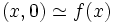$(x,0) \simeq f(x)$ and$(x,1) \simeq g(x)$.

## More specific constructions

Specific cases of the above arise either by setting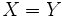$X = Y$ and$f$ the identity map (or correspondingly for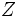$Z$ and$g$) or setting$Y$ or$Z$ to be a one-point space. If we impose only one constraint, the resultant construction is the construction corresponding to the other unspecified map. If we impose two constraints, then the resulting construction depends only on the input space$X$.

Note that the roles of$Y$ and$Z$ can be interchanged here.

Name of construction One-point spaces? Identity maps? Remaining input? Conclusion
mapping cylinder --$f$, so$X = Y$$g:X \to Z$ With this stipulation, the double mapping cylinder is the same as the mapping cylinder for$g:X \to Z$.
mapping cone$Z$, so$g$ sends all of$X$ to one point --$f:X \to Y$ With this stipulation, the double mapping cylinder is the same as the mapping cone for$f:X \to Y$.
cone space$Z$, so$g$ sends all of$X$ to one point$f$, so$X = Y$ Only the space$X$ With this stipulation, we get the cone space for$X$
suspension both$Y$ and$Z$ -- Only the space$X$ With this stipulation, we get the suspension of$X$
cylinder -- both$f$ and$g$ only the space$X$ With this stipulation, we get the cylinder on$X$, i.e., the product of$X$ and the unit interval

### The join

The join of two spaces$A$ and$B$ can be constructed as a double mapping cylinder as follow: Set$X = A \times B$,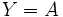$Y = A$ and$Z = B$, and let$f,g$ be the coordinate projection maps.

## Facts

There is a relation between the homology of the double mapping cylinder of$f$ and$g$, and the homologies of the spaces$X$,$Y$ and$Z$. The relation is described by the exact sequence for double mapping cylinder.